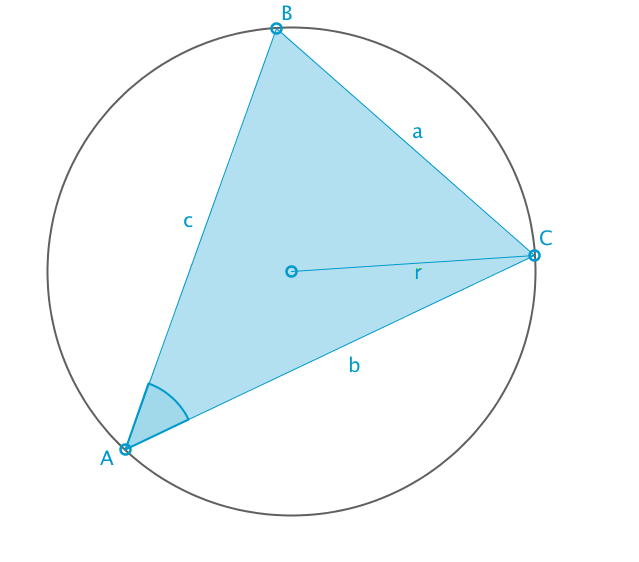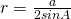# Project: Circumscribed Triangle Radius Problem

The triangle in the figure below is circumscribed by a circle with radius.Find the value of.

Any triangle can be circumscribed by a circle.Find a formula for the radius of the circle, given the three sides of the triangle.

Show that, if you haven’t already done so, that.

Find a similar expression forusing angleand side; then angleand side. Hence prove the sine rule.

Done here! Take me back to the triangles menu.